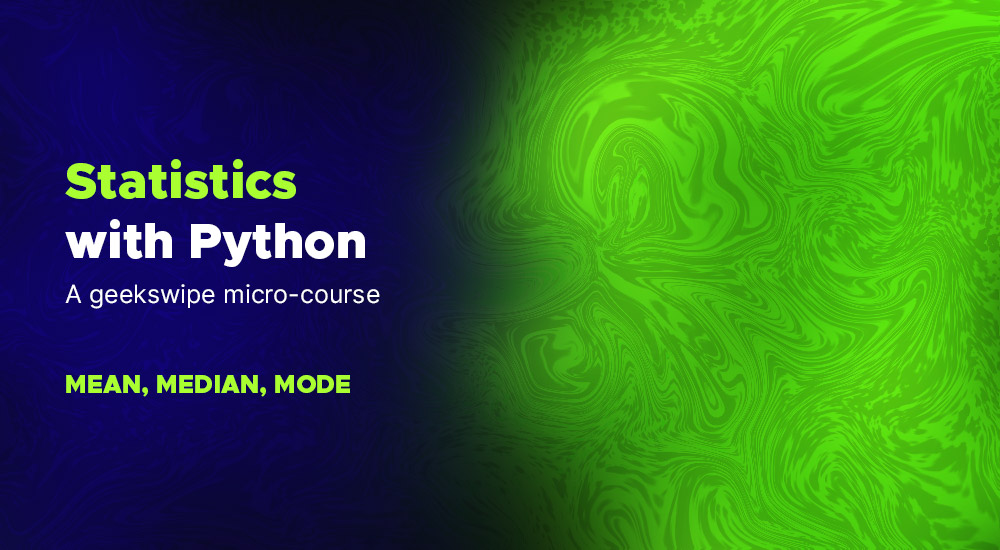# Statistics with Python – Mean, Median, and ModeIf you are into mathematical thinking or stuck in a boring statistics class, this Geekswipe statistics series is for you. I hope it will perk up your sessions with quick and easy micro-lessons on statistics with Python.

As an engineering student and a developer, I use statistics mostly around the domain of academic data analysis and visualization. So this is clearly a top-down approach, more aligned with the tools like Pandas, Numpy, and Scikit-learn, with simple and easy crash courses and explainers on statistical concepts.

And most of the lessons here are based on my drafted posts from my college days. New lessons might take some time. And a few examples might not have syntax highlighting and stuff. Once everything is streamlined, you can expect an index of all the lessons here.

## Quick crash course on statistics

Let’s start with the basics. Let’s say you have a huge dataset at your hand. Statistics is how you communicate that data. It’s how you express what the data represents. In other words, you summarise or visualize the data so it’s easy to communicate with others. This business of visualizing the data and drawing actionable intelligence from it is called statistics.

The ‘visualizing data’ part is called descriptive statistics. The ‘drawing intelligence from the visualized data’ part is called inferential statistics.

## Descriptive statistics

Let’s start with descriptive statistics. You can describe a data based on its measure of central tendency or a measure of dispersion or spread.

## Measure of central tendency

In this micro lesson, we’ll look at the three common measures of central tendency—the mean, median, and mode.

• Mean – The average of the given set of values.
• Median – The value in the middle when you arrange the given set of value in ascending order.
• Mode – The value that occurs frequently in the given set.

## Examples with Python

At the time of this writing, Python 3 did not have the native `statistics` library. I have used `numpy` here and probably it’s best to use it—you’ll end up using it anyway for multi-dimensional arrays.

### Mean

``````import numpy as np

numbers = np.array([20, 34, 21, 18, 22, 21, 45, 10, 14, 20])
mean = np.mean(numbers)

print(mean)
``````

The output will be `22.5`, which is the average of all the values in the list `scores`. Now, this is a one-dimensional array. For two dimensional array, you’d need to mention the axis along which you need to calculate the mean. Refer numpy documentation for more examples.

### Median

``````import numpy as np

numbers = np.array([20, 34, 21, 18, 22, 21, 45, 10, 14, 20])
median = np.median(numbers)

print(median)
``````

The result will be `20.5`. This is the middle value you get when you arrange the scores in ascending order. If the number of values in a list is odd, the middle value will be its median. In the case of even counts, the two middle values are averaged like in the above example.

### Mode

``````import numpy as np
from scipy import stats

numbers = np.array([20, 34, 21, 18, 22, 21, 45, 10, 14, 20])
mode = stats.mode(numbers)

print(mode)
``````

The result will be `(array([ 20.]), array([ 2.]))`, which means `20` is the mode (a value that occurs most often in the list) and `2` is the count of the occurrence.

Wait! `21` occurs twice too. Well, you’re right there, champ! it is a mode as well. Except that this library shows us the first encountered mode alone.

In our next lesson, we’ll explore the various statistical methods of measure of dispersion and look at some python examples on that. But with my semesters coming up, it might take a while for me to come up with new lessons. Happy coding until then!

This post was first published on July 12, 2012.Karthikeyan KC

Aeronautical engineer, dev, science fiction author, gamer, and an explorer. I am the creator of Geekswipe. I love writing about physics, aerospace, astronomy, and python. I created Swyde. Currently working on Arclind Mindspace.

### 2 Responses

1.Arun

•Karthikeyan KC